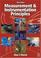### Methods of Measurement

Data is displayed for academic year: 2022./2023.

#### Course Description

Accuracy of measurement, errors and the expression of uncertainties. Passive elements of measurement circuits. Analog and digital measuring instruments. The main properties, limitations, accuracy and applications. Measurements of voltage and current. Voltmeters and ammeters. Compensators and calibrators, high voltage dividers. Conventional and unconventional measuring transformers. Thermal current comparator. Resistance measurement. I-U method, measurement of insulation resistance and ground resistance. Measurement of capacity and inductance. Measuring bridges, digital impedance and admittance instruments. Oscilloscopes. Sampling of signals. Application of an oscilloscope. Measuring probe. Power measurement. DC, AC single phase and three phase measurements. Digital wattmeter. Semi-direct and indirect power measurement. Measurement of reactive and apparent power. Measurement of energy. Watthour meters; tariff setting, remote reading. Quality of electric energy. Measuring converters. Characteristics, the normalized output values, application. Temperature measurements. Resistive sensors, thermocouples. Measurement of pressure, flow, strain and relative extension. Application of unbalanced bridges. Protection from interference.

#### Study Programmes

[FER2-HR] Electrical Power Engineering - module
Elective Courses (6. semester)

#### General Competencies

The course gives the basic knowledge about the principles of operation of measuring instruments and various methods for measuring different electrical and non-electrical quantities. The students will have theoretical basis and practical skills to implement modern measuring instruments in many different methods, and ability to analyse the obtained results of measurements.

#### Learning Outcomes

1. choose measuring instruments with respect to the measurement requirements
2. apply appropriate measurement procedure for a given measurand
3. identify influential factors and make the correction of the results
4. practice correctly measuring instruments
5. classify systematic and random errors in the measurement procedure
6. show measurement result and the corresponding measurement uncertainty

#### Forms of Teaching

Lectures

Lectures are designed as 10 thematic units that are logically continue each other. Following the lectures, students are introduced into the basics of quality theory and the issues of systematic quality management.

Exams

Examination is conducted through mid-term exam and final exam.

Continuous Assessment Exam
Laboratory Exercises 0 % 30 % 0 % 30 %
Mid Term Exam: Written 0 % 20 % 0 %
Final Exam: Written 0 % 50 %
Exam: Written 0 % 50 %
Exam: Oral 20 %

#### Week by Week Schedule

1. Accuracy of measurement and theory of errors. Standard deviation and statistical analysis of results. Measurement uncertainty, type A and type B. The complex expression of uncertainty and uncertainty. Computational examples.
2. Passive elements of measurement circuits. Real parameters and the equivalent diagrams. Parasitic parameters and frequency dependent parameters. Waveforms of AC quantities, definition and calculation of mean and effective values. Computational examples.
3. Analog and digital measuring instruments. The principle of operation, characteristics, limitations and accuracy. Application of analog and digital multimeter. Computational examples.
4. Measurement of direct currents. Ammeters. Current clamp with a Hall probe. DC current transformer.
5. Measurement of DC voltage. The compensation method and method of frequency transposition. Measuring amplifiers. High voltage divider and measuring probes. Electrostatic voltmeter.
6. Measurement of alternating current. Instruments with rectifiers. Measurement via thermoconverters. Conventional and unconventional current transformers.
7. Measurement of AC voltages. Voltmeters responsive to the effective value. Conventional and capacitive voltage transformer. Inductive divider. Ball spark gap and Chubb procedure.
8. Mid-term exam
9. Mid-term exam
10. Resistance measurement. UI method and comparison method. Ohmmeter method. Constant current method. Method of charge loss. Measuring the resistance of insulating materials. Measurement of ground resistance.
11. Measurement of inductance and capacitance. UI methods. Resonance method. Measuring bridges: Maxwell and Wien bridge. Schering and Glynn bridge. Digital RLC bridges.
12. Power measurement. DC, AC single phase and three phase metering. Digital wattmeter. Semi-direct and indirect measurement of power. Reactive and apparent power.
13. Measurement of energy. Watthour meters. Additional control functions: charging, remote sensing. The quality of electrical energy.
14. Measuring transducers. Characteristics, the normalized output values, application. Temperature measurements. Resistive sensors, thermocouples. Measurement of pressure, flow, stress and relative displacement. The application of unbalanced bridges.
15. Magnetic measurements

#### Literature

V. Bego (2003.), Mjerenja u elektrotehnici, GraphisA. Morris (2001.), Measurement and Instrumentation Principles, Butterworth-Heinemann
V. Haasz, M. Sedláček (1998.), Electrical Measurements. Instruments and Methods, VČVUT, Praha
D. Vujević, B. Ferković (2001.), Osnove elektrotehničkih mjerenja, I. i II. dio, Školska knjiga

#### General

ID 34334
Summer semester
4 ECTS
L0 English Level
L1 e-Learning
30 Lectures
0 Seminar
0 Exercises
15 Laboratory exercises
0 Project laboratory